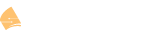# How to create Complex Wildcard Masks

Thank you for this great explanation.
Make much more sense now19 posts were merged into an existing topic: How to create Complex Wildcard Masks

Hi Rene ,

Great article everHow i will filter only odd ip from the block 192.168.0.0/24 and allow Even IP from this ??

br//
zaman

The simplest way (which permits only even IPs from 192.168.0.0/24) would be this:

``````ip access-list standard ACL_PERMIT-EVEN
permit 192.168.0.0 0.0.0.254
``````

Remember that ACLs have an implicit deny all at the end, so nothing other than what is matched would be allowed. If you wanted to let everything through except the odd IPs from 192.168.0.0/24, you would have to tweak this a bit:

``````ip access-list standard ACL_DENY-ODD
deny 192.168.0.1 0.0.0.254
permit any
``````

--Andrew

Hi Rene,

When find the wildcard mask for the Even subnet you mentioned:
'The first two octets are the same for all the subnets so we use all zeroes for the wildcard mask. In the third octet we use a 1 (don’t care) for all bits except for the 8th bit…it has to match. ’

Use 1 for all bits except the 8th bit (which is 0) will produce 1111 1110 which gives you a wildcard mask of 0.0.254.255.
Why is it that (except the 8th bit) doesn’t apply to Uneven subnet where the 8th bit is 1? As Uneven subnet also uses wildcard mask of 0.0.254.255,

Hello Kenneth.

Using the same example as that found in the lesson, if you want to specify only the EVEN subnets, then you will use a network address of 192.168.0.0 and a wildcard mask of 0.0.254.255. Note the following:

``````192.168.0.0   11000000	10101000	00001000	00000000
0.0.254.255   00000000	00000000	11111110	11111111
``````

The last bit of the third octet of the network address must remain 0 since the wildcard mask has a 0 in that position. If that remains 0, then all the values of the third octet will be EVEN, such as 0, 2, 4, 6, 8, 10 and so on.

Alternatively, if you want to specify only the ODD subnets, you will still use the same wildcard mask, BUT, you will use a different subnet address. In this case you would use 192.168.1.0 and a wildard mask of 0.0.254.255. Note the following:

``````192.168.1.0   11000000	10101000	00001001	00000000
0.0.254.255   00000000	00000000	11111110	11111111
``````

In this case, the last bit of the third octet of the network address must remain 1 since the wildcard mask has a 0 in that position. If that remains 1, then all the values of the third octet will be ODD, such as 1, 3, 5, 7, 9, 11 and so on.

So it is the initial subnet address that indicates whether it is the ODD or EVEN subnets that will be specified and not only the wildcard mask itself.

I hope this has been helpful!

Laz